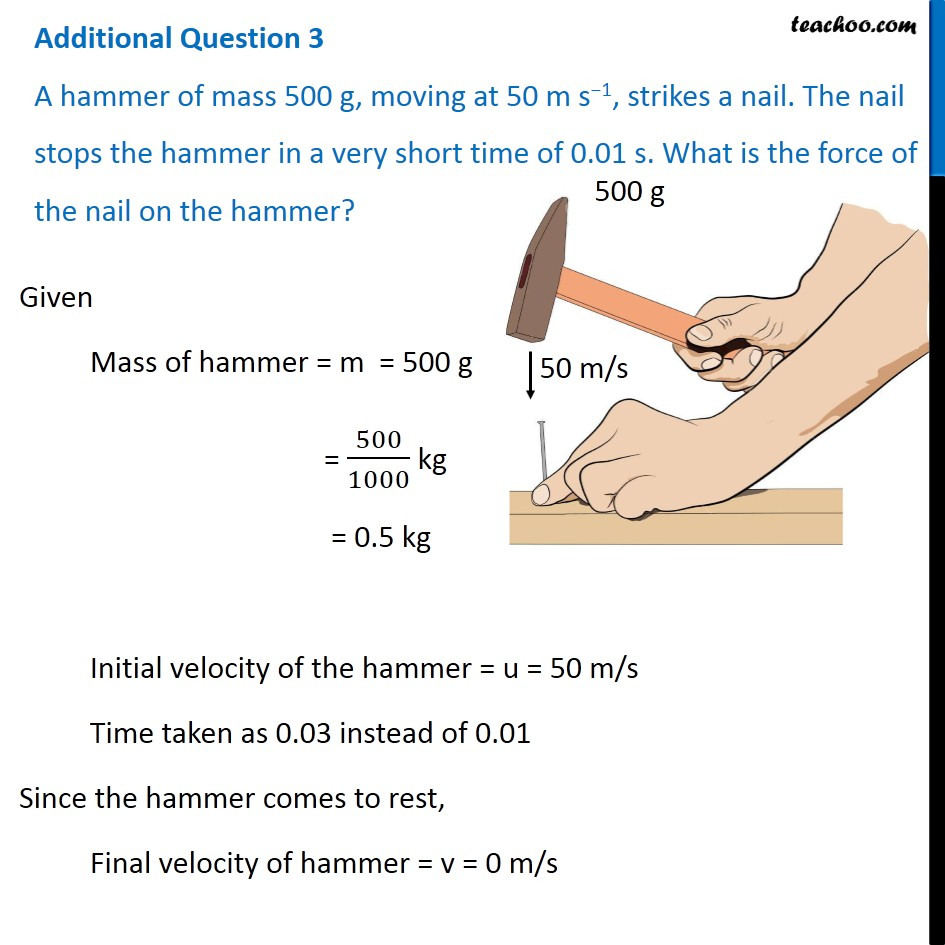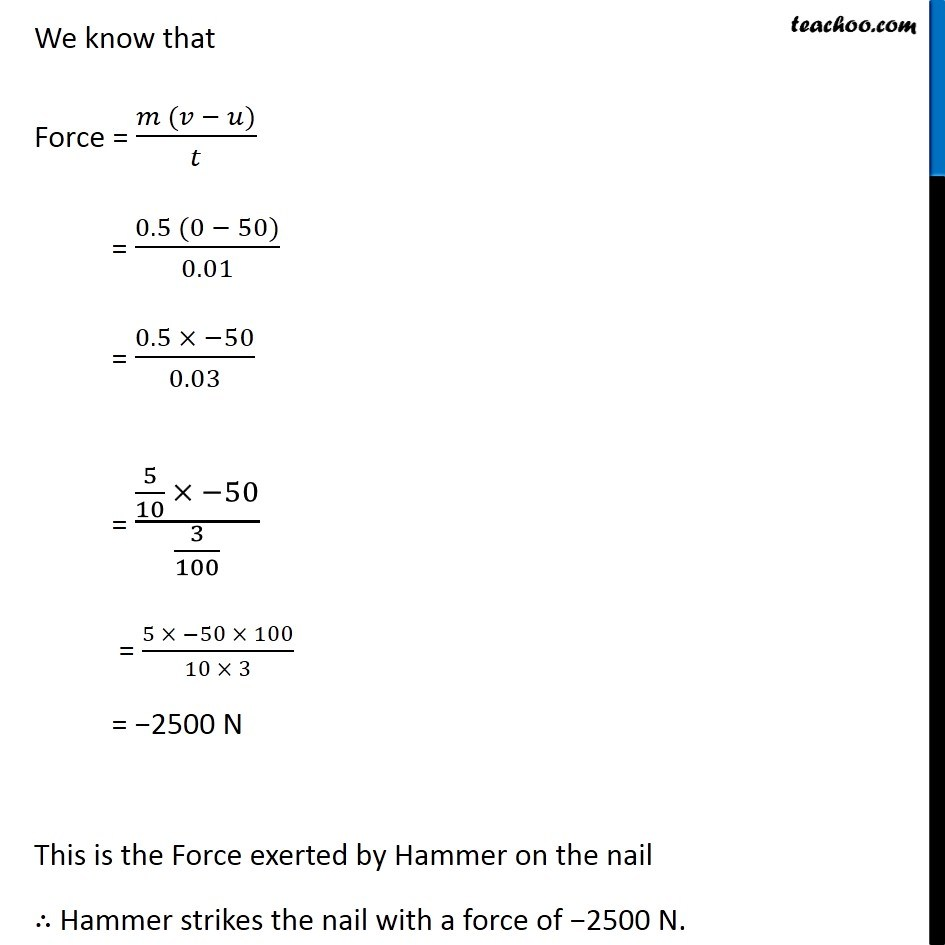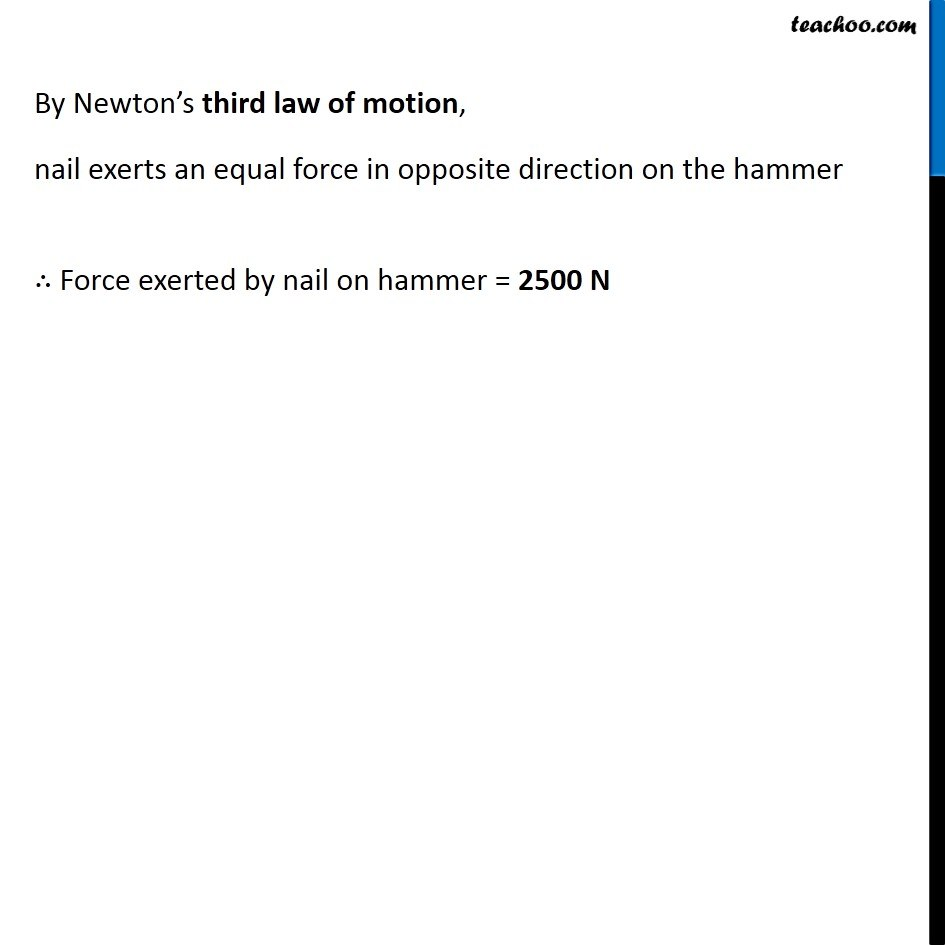Class 9
Chapter 9 Class 9 - Force and Laws Of MotionLearn in your speed, with individual attention - Teachoo Maths 1-on-1 Class

### Transcript

Additional Question 3 A hammer of mass 500 g, moving at 50 m s−1, strikes a nail. The nail stops the hammer in a very short time of 0.01 s. What is the force of the nail on the hammer? Given Mass of hammer = m = 500 g = 500/1000 kg = 0.5 kg Initial velocity of the hammer = u = 50 m/s Time taken as 0.03 instead of 0.01 Since the hammer comes to rest, Final velocity of hammer = v = 0 m/s We know that Force = (𝑚 (𝑣 − 𝑢))/𝑡 = (0.5 (0 − 50))/0.01 = (0.5 × −50)/0.03 = (5/10 × −50)/(3/100) = (5 × −50 × 100)/(10 × 3) = −2500 N This is the Force exerted by Hammer on the nail ∴ Hammer strikes the nail with a force of −2500 N. By Newton’s third law of motion, nail exerts an equal force in opposite direction on the hammer ∴ Force exerted by nail on hammer = 2500 N